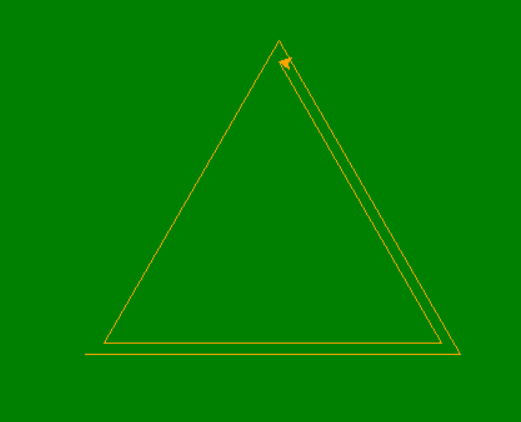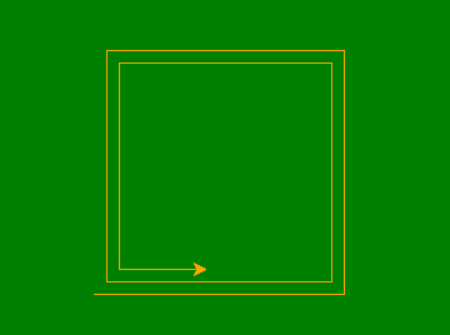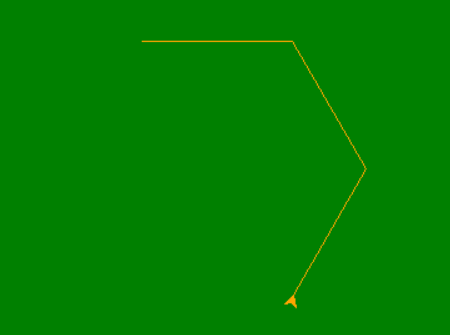# Draw Shape inside Shape in Python Using Turtle

Prerequisites: Turtle Programming in Python

Turtle is a Python feature like a drawing board, which let us command a turtle to draw all over it! We can use many turtle functions which can move the turtle around. Turtle comes in the turtle library. The turtle module can be used in both object-oriented and procedure-oriented ways.

Some of the commonly used methods which are also used here are:

• forward(length): moves the pen in the forward direction by x unit.
• backward(length): moves the pen in the backward direction by x unit.
• right(angle): rotate the pen in the clockwise direction by an angle x.
• left(angle): rotate the pen in the anticlockwise direction by an angle x.
• penup(): stop drawing of the turtle pen.
• pendown(): start drawing of the turtle pen.

In this article, we will draw various shape inside the similar shape like drawing traingles inside triangle.

## Triangle inside Triangle

• Define an instance for turtle.
• For a square execute a loop 3 times (sides).
• In every iteration move turtle 120 units forward.
• This will make up a Triangle.
• This is made multiple times to form triangles inside triangle using a function.

Below is the python implementation.

## Python3

 `# import the turtle modules  ` `import` `turtle  ` ` `  ` `  `# define the function ` `# for triangle ` `def` `form_tri(side): ` `    ``for` `i ``in` `range``(``3``): ` `        ``my_pen.fd(side) ` `        ``my_pen.left(``120``) ` `        ``side ``-``=` `10` ` `  `         `  `# Forming the window screen ` `tut ``=` `turtle.Screen() ` `tut.bgcolor(``"green"``) ` `tut.title(``"Turtle"``) ` ` `  `my_pen ``=` `turtle.Turtle() ` `my_pen.color(``"orange"``) ` ` `  `tut ``=` `turtle.Screen()            ` ` `  `# for different shapes ` `side ``=` `300` `for` `i ``in` `range``(``10``): ` `    ``form_tri(side) ` `    ``side ``-``=` `30`

Output :## Square inside Square

• Define an instance for turtle.
• For a square execute a loop 4 times (sides).
• In every iteration move turtle 90 units forward.
• This will make up a Square.
• This is made multiple times to form squares inside square using a function.

Below is the python implementation.

## Python3

 `# import the turtle modules  ` `import` `turtle  ` ` `  `# define the function ` `# for square ` `def` `form_sq(side): ` `    ``for` `i ``in` `range``(``4``): ` `        ``my_pen.fd(side) ` `        ``my_pen.left(``90``) ` `        ``side ``-``=` `5` ` `  `         `  `# Forming the window screen ` `tut ``=` `turtle.Screen() ` `tut.bgcolor(``"green"``) ` `tut.title(``"Turtle"``) ` ` `  `my_pen ``=` `turtle.Turtle() ` `my_pen.color(``"orange"``) ` ` `  `tut ``=` `turtle.Screen()            ` ` `  `# for different shapes ` `side ``=` `200` ` `  `for` `i ``in` `range``(``10``): ` `    ``form_sq(side) ` `    ``side``-``=` `20`

Output :## Hexagon inside Hexagon

• Define an instance for turtle.
• For a hexagon execute a loop 6 times (sides).
• In every iteration move turtle 300 units forward.
• This will make up a Hexagon.
• This is made multiple times to form hexagons inside hexagon using a function.

Below is the python implementation.

## Python3

 `# import the turtle modules ` `import` `turtle ` ` `  ` `  `# define the function ` `# for hexagon ` `def` `form_hex(side): ` `    ``for` `i ``in` `range``(``6``): ` `        ``my_pen.fd(side) ` `        ``my_pen.left(``300``) ` `        ``side ``-``=` `2` ` `  ` `  `# Forming the window screen ` `tut ``=` `turtle.Screen() ` `tut.bgcolor(``"green"``) ` `tut.title(``"Turtle"``) ` ` `  `my_pen ``=` `turtle.Turtle() ` `my_pen.color(``"orange"``) ` ` `  `tut ``=` `turtle.Screen() ` ` `  `# for different sizes ` `side ``=` `120` ` `  `for` `i ``in` `range``(``5``): ` `    ``form_hex(side) ` `    ``side ``-``=` `12`

Output :My Personal Notes arrow_drop_upCheck out this Author's contributed articles.

If you like GeeksforGeeks and would like to contribute, you can also write an article using contribute.geeksforgeeks.org or mail your article to contribute@geeksforgeeks.org. See your article appearing on the GeeksforGeeks main page and help other Geeks.

Please Improve this article if you find anything incorrect by clicking on the "Improve Article" button below.

Article Tags :

Be the First to upvote.

Please write to us at contribute@geeksforgeeks.org to report any issue with the above content.